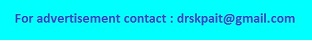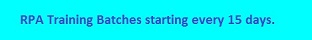# Hooke's Law Calculator

Hooke's Law of elasticity is an approximation that states that the amount by which a material body is deformed (the strain) is linearly related to the force causing the deformation (the stress).
Force : Fx=-k(x-xo), Spring Force Constant : k=Fx/(x-xo), Distance from Equilibrium : xo=(Fx/k)+x, Spring Equilibrium Position : x=xo-(fx/h) where, Fx = Force, k = Spring Force Constant, x =Distance from Equilibrium, x0 = Spring Equilibrium Position.
 Calaculate Force(Fx) Spring Force Constant(k) Distance from Equilibrium(x) Spring Equilibrium Position(xx) Spring Force Constant(k): N/m Distance from Equilibrium(x): m Spring Equilibrium Position(x0): m Force(Fx): N